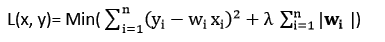# Regression Analysis in Machine learning

In this page, we will learn What is Regression analysis?, Terminologies Related to the Regression Analysis, Why do we use Regression Analysis?, Types of Regression, Linear Regression, Logistic Regression, Polynomial Regression, Support Vector Regression, Decision Tree Regression, Ridge Regression, Lasso Regression.

## What is Regression analysis?

Regression analysis is a statistical method for modeling the connection between one or more independent variables and a dependent (target) variable. Regression analysis, in particular, allows us to see how the value of the dependent variable changes in relation to an independent variable while the other independent variables are maintained constant. Temperature, age, salary, price, and other continuous/real values are predicted.

The following example will help us grasp the notion of regression analysis: Assume there is a marketing firm A that produces a variety of advertisements each year and generates revenue from them. The below list shows the advertisement made by the company in the last 5 years and the corresponding sales:

\$90 \$100
\$120 \$1300
\$150 \$1800
\$100 \$1200
\$130 \$1380
\$200 ??

Now, the corporation wants to run a \$200 advertisement in 2019 and wants to know what the sales forecast is for that year. Regression analysis is required to handle such prediction problems in machine learning.

Regression is a supervised learning technique that aids in the discovery of variable correlations and allows us to forecast a continuous output variable using one or more predictor variables. Prediction, forecasting, time series modeling, and identifying the causal-effect link between variables are all common applications.

We construct a graph connecting the variables that best fits the given datapoints in regression, and the machine learning model may make predictions about the data using this plot. "Regression shows a line or curve that passes through all the datapoints on target-predictor graph in such a way that the vertical distance between the datapoints and the regression line is minimum." A model's ability to capture a strong link is determined by the distance between datapoints and line.

The following are some examples of regression:

• Prediction of rain using temperature and other factors
• Determining Market trends
• Prediction of road accidents due to rash driving.

## Terminologies Related to the Regression Analysis:

Dependent Variable: The dependent variable is the main factor in regression analysis that we wish to predict or understand. It's also known as a target variable.

Independent Variable: An independent variable, often known as a predictor, is a factor that affects the dependent variables or is used to predict the values of the dependent variables.

Outliers: An outlier is a value that is either extremely low or extremely high in relation to other observed values. An outlier can skew the results, thus it's best to avoid them.

Multicollinearity: It is a circumstance in which the independent variables are more highly associated with each other than the other variables. It shouldn't be in the dataset because it messes up the ranking of the most important variable.

Underfitting and Overfitting: Overfitting occurs when our method performs well on the training dataset but not on the test dataset. Underfitting is a problem that occurs when our method does not perform well even with a training dataset.

## Why do we use Regression Analysis?

Regression analysis, as previously said, aids in the prediction of a continuous variable. In the real world, there are a variety of scenarios where we need to make future predictions, such as weather conditions, sales forecasts, marketing trends, and so on. In these cases, we need technology that can create more accurate predictions. In such a circumstance, regression analysis, a statistical tool utilized in machine learning and data science, is required. Regression analysis can also be used for the following reasons:

• The relationship between the target and the independent variable is estimated using regression.
• It's used to look for patterns in data.
• It aids in the prediction of real and continuous variables.
• We can confidently establish the most important factor, the least important element, and how each factor affects the other ones by using regression.

## Types of Regression

In data science and machine learning, there are many different forms of regressions. Each type has its own significance in different settings, but all regression methods assess the effect of the independent variable on dependent variables at their core. We'll go over some of the most common types of regression in this section:

• Linear Regression
• Logistic Regression
• Polynomial Regression
• Support Vector Regression
• Decision Tree Regression
• Random Forest Regression
• Ridge Regression
• Lasso Regression

## Linear Regression:

• Linear regression is a predictive analysis tool based on statistical regression.
• It is one of the most basic and straightforward algorithms for calculating regression and displaying the relationship between continuous variables.
• It is used in machine learning to solve the regression problem.
• Linear regression, as the name implies, depicts a linear relationship between the independent variable (X-axis) and the dependent variable (Y-axis).
• Simple linear regression is defined as linear regression with only one input variable (x). When there are several input variables, the linear regression is referred to as multiple linear regression.
• The graphic below depicts the relationship between variables in the linear regression model. We're estimating an employee's wage based on his or her year of experience.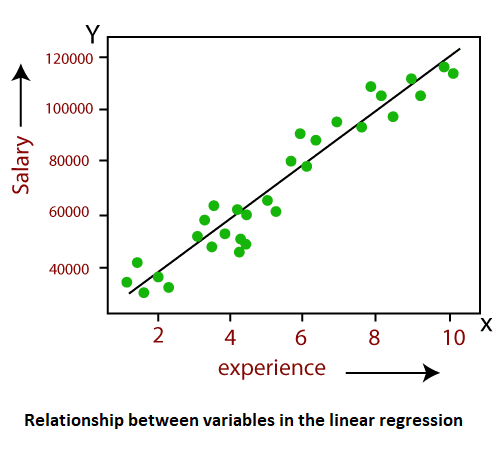• The mathematical equation for Linear regression is below:

### Y = aX + b

Here, Y = dependent variables (target variables),
X= Independent variables (predictor variables),
a and b are the linear coefficients

Some popular applications of linear regression are:

• Analyzing trends and sales estimates
• Salary forecasting
• Real estate prediction
• Arriving at ETAs in traffic.

## Logistic Regression:

• Another supervised learning approach for solving classification problems is logistic regression. We have binary or discrete dependent variables in classification problems, such as 0 or 1.
• The categorical variables used in the logistic regression algorithm are 0 or 1, Yes or No, True or False, Spam or non spam, and so on.
• It is a predictive analysis technique that is based on the probability notion.
• Although logistic regression is a sort of regression, it differs from linear regression in terms of how it is employed.
• The sigmoid function, often known as the logistic function, is a sophisticated cost function used in logistic regression. In logistic regression, this sigmoid function is used to model the data. The following is a representation of the function: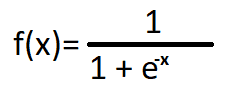• f(x)= Output between the 0 and 1 value.
• x= input to the function.
• e= base of natural logarithm.

When we provide the input values (data) to the function, it gives the S-curve as follows: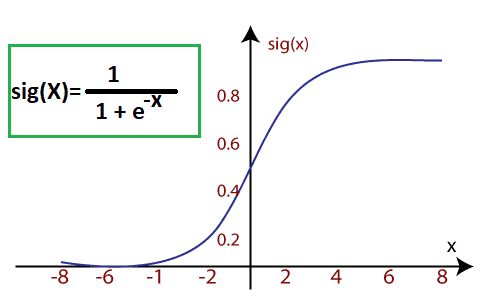It employs the concept of threshold levels, with numbers above the threshold level being rounded to 1 and below the threshold level being rounded to 0.

Logistic regression can be divided into three categories:

• Binary(0/1, pass/fail)
• Multi(cats, dogs, lions)
• Ordinal(low, medium, high)

## Polynomial Regression:

• Polynomial regression is a sort of regression that uses a linear model to model a non-linear dataset.
• It works in the same way as multiple linear regression, except it fits a non-linear curve between the value of x and the conditional values of y.
• If a dataset contains datapoints that are distributed in a non-linear pattern, linear regression will not provide the best fit for those datapoints. Polynomial regression is required to cover such data points.
• The original features are translated into polynomial features of a certain degree and then modeled using a linear model in polynomial regression. This signifies that a polynomial line is the best match for the data points.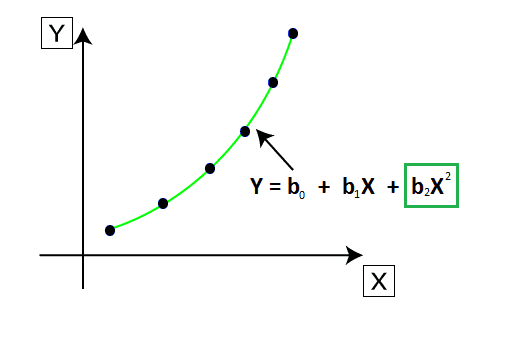• The equation for polynomial regression also derived from linear regression equation that means Linear regression equation Y = b0 + b1x, is transformed into Polynomial regression equation Y = b0+b1x+ b2x2+ b3x3+.....+ bnxn.
• Here Y is the predicted/target output, b0, b1,... bn are the regression coefficients. x is our independent/input variable.
• Because the coefficients are still linear with quadratics, the model is still linear.

Note: Polynomial regression differs from Numerous Linear regression in that a single element has different degrees rather than multiple variables with the same degree.

## Support Vector Regression:

• The Support Vector Machine (SVM) is a supervised learning technique that may be used to solve both regression and classification issues. Support Vector Regression is the name given to it when it is used to solve regression problems.
• Support Vector Regression (SVR) is a continuous-variable regression algorithm. The following are some of the terms used in Support Vector Regression:
• Kernel: A kernel is a function that maps lower-dimensional data to higher-dimensional data.
• Hyperplane: In SVM, it is a line that separates two classes, but in SVR, it is a line that aids in the prediction of continuous variables and covers the majority of datapoints.
• Boundary line: Aside from the hyperplane, boundary lines are the two lines that establish a margin for datapoints.
• Support vectors: These are the datapoints that are closest to the hyperplane and have the opposite class.
• In SVR, we always strive to find a hyperplane with the largest possible margin, so that the maximum number of datapoints are covered. The main goal of SVR is to consider the maximum datapoints within the boundary lines and the hyperplane (best-fit line) must contain a maximum number of datapoints. Consider the below image: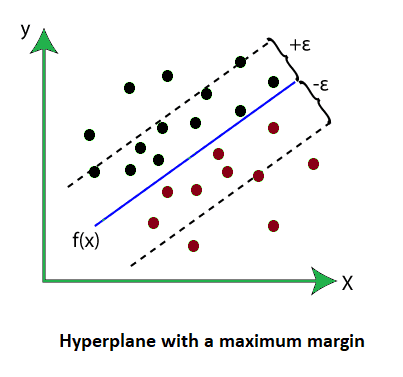Here, the blue line is called hyperplane, and the other two lines are known as boundary lines.

## Decision Tree Regression:

• The Decision Tree algorithm is a supervised learning system that can solve both classification and regression problems.
• It can handle both categorical and numerical data to answer problems.
• Each internal node represents the "test" for an attribute, each branch indicates the test's outcome, and each leaf node represents the final choice or conclusion.
• Starting with the root node/parent node (dataset), a decision tree is built that splits into left and right child nodes (subsets of dataset). These child nodes are further subdivided into their children nodes, with the parent node of those nodes becoming the parent node. Take the case of the below: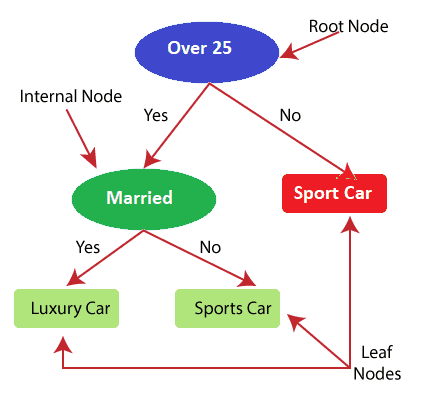• The model is attempting to forecast a person's choice between Sports automobiles and Luxury cars in the above image of Decision Tee regression.
• Random forest is an extremely effective supervised learning algorithm that can do both regression and classification problems.
• Random Forest regression is an ensemble learning method that mixes many decision trees and predicts the final outcome using the average of each tree's output. The combined decision trees are referred to as base models, and they may be written out as:
g(x)= f0(x)+ f1(x)+ f2(x)+....
• Random forest employs the Bagging or Bootstrap Aggregation ensemble learning technique, in which aggregated decision trees run in parallel and do not interact.
• We can avoid Overfitting in the model by using Random Forest regression to create random subsets of the dataset.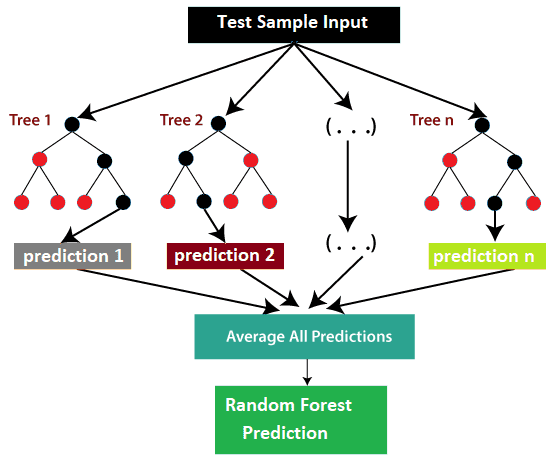## Ridge Regression:

• Ridge regression is one among the foremost sturdy versions of statistical regression during which a little quantity of bias is introduced so we are able to convalesce long run predictions.
• The amount of bias additional to the model is thought as Ridge Regression penalty. we are able to cypher this penalty term by multiplying with the lambda to the square weight of every individual options.
• The equation for ridge regression can be: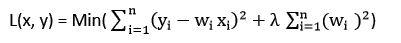• Ridge regression is a regularization technique, which is used to reduce the complexity of the model as well as It is also called as L2 regularization.
• It helps to unravel the issues if we've got additional parameters than samples.

## Lasso Regression:

• Lasso regression is another regularization technique to scale back the complexness of the model.
• It is just like the Ridge Regression except that penalty term contains solely absolutely the weights rather than a sq. of weights.
• Since it takes absolute values, hence, it will shrink the slope to zero, whereas Ridge Regression will solely shrink it with reference to zero.
• It is additionally known as as L1 regularization. The equation for Lasso regression can be: